### Showing:

 Annotations Diagrams Properties Source Used by
Element gml:FormulaType / gml:a
Namespace http://www.opengis.net/gml
Diagram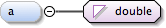Type double
Properties
 content: simple minOccurs: 0
Source
 ``
Schema location http://schemas.opengis.net/gml/3.1.1/base/units.xsd
Element gml:FormulaType / gml:b
Namespace http://www.opengis.net/gml
Diagram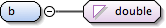Type double
Properties
 content: simple
Source
 ``
Schema location http://schemas.opengis.net/gml/3.1.1/base/units.xsd
Element gml:FormulaType / gml:c
Namespace http://www.opengis.net/gml
Diagram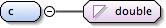Type double
Properties
 content: simple
Source
 ``
Schema location http://schemas.opengis.net/gml/3.1.1/base/units.xsd
Element gml:FormulaType / gml:d
Namespace http://www.opengis.net/gml
Diagram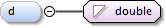Type double
Properties
 content: simple minOccurs: 0
Source
 ``
Schema location http://schemas.opengis.net/gml/3.1.1/base/units.xsd
Complex Type gml:FormulaType
Namespace http://www.opengis.net/gml
Annotations
 `Paremeters of a simple formula by which a value using this unit of measure can be converted to the corresponding value using the preferred unit of measure. The formula element contains elements a, b, c and d, whose values use the XML Schema type "double". These values are used in the formula y = (a + bx) / (c + dx), where x is a value using this unit, and y is the corresponding value using the preferred unit. The elements a and d are optional, and if values are not provided, those parameters are considered to be zero. If values are not provided for both a and d, the formula is equivalent to a fraction with numerator and denominator parameters.`
Diagram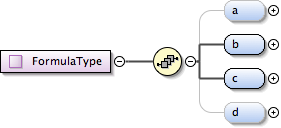Used by
 Element gml:ConversionToPreferredUnitType/gml:formula
Model gml:a{0,1} , gml:b , gml:c , gml:d{0,1}
Children gml:a, gml:b, gml:c, gml:d
Source
 ``` Paremeters of a simple formula by which a value using this unit of measure can be converted to the corresponding value using the preferred unit of measure. The formula element contains elements a, b, c and d, whose values use the XML Schema type "double". These values are used in the formula y = (a + bx) / (c + dx), where x is a value using this unit, and y is the corresponding value using the preferred unit. The elements a and d are optional, and if values are not provided, those parameters are considered to be zero. If values are not provided for both a and d, the formula is equivalent to a fraction with numerator and denominator parameters. ```
Schema location http://schemas.opengis.net/gml/3.1.1/base/units.xsd